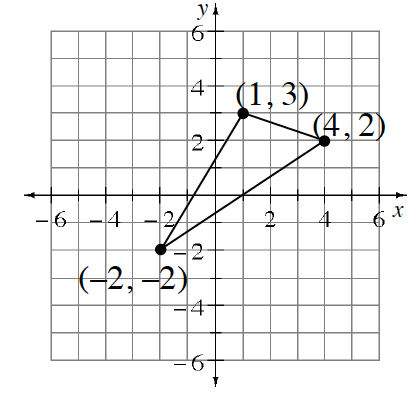### Home > ACC7 > Chapter 6 Unit 7 > Lesson CC3: 6.2.2 > Problem6-58

6-58.

Create a large coordinate graph on graph paper and graph the triangle at right. Multiply the $y$-coordinate of each point by $4$. Then graph the new shape. Make sure you connect your points. List the points for the new shape. Are the two figures similar? Why or why not?

Similar triangles have equal corresponding angles. That is, they should be the same shape.Explore the problem using the eTool below.
Click the link at right for the full version of the eTool: